### Home > CALC > Chapter 6 > Lesson 6.4.1 > Problem6-130

6-130.
1. Trace the distance-time graph and the tangent at t = 1 second on your paper. Distance d is measured in feet. Homework Help ✎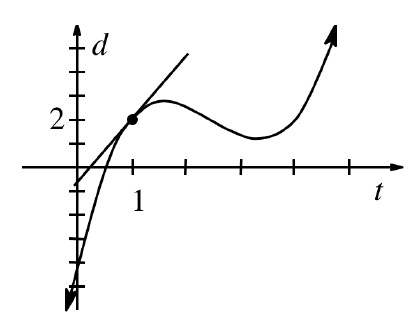1. Estimate the velocity at t = 1 sec.

2. Find another time on the graph where the object has the same velocity. Show on your graph how you know the velocities are equal.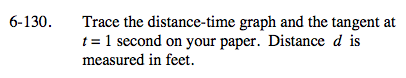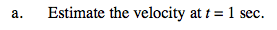Estimate the slope of the tangent.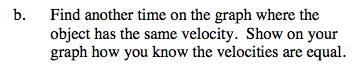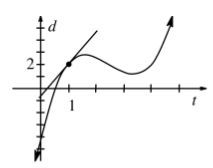Sketch various tangent lines to the graph of d(t). Keep sketching until you find one with the same slope as the tangent at t = 1.

Parallel lines have the same slope.